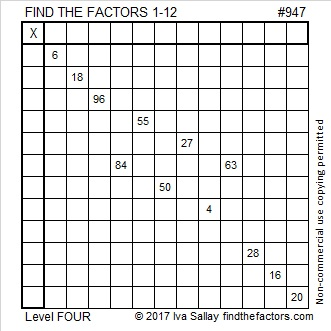# 947 and Level 4

If you know how to multiply and divide, then you can solve this puzzle. Just use logic to find the factors from 1 to 12 that go in the first column and the top row. Go ahead give it a try!Print the puzzles or type the solution in this excel file: 12 factors 942-950

Now here’s a little about the number 947:

947 is a prime number that can be written as the sum of seven consecutive prime numbers:
113 + 127 + 131 + 137 + 139 + 149 + 151 = 947

947 is a palindrome in three other bases:
3B3 BASE 16 (B is 11 base 10), because 3(16²) + 11(16¹) + 3(16⁰) = 947
232 BASE 21 because 2(21²) + 3(21¹) + 2(21⁰) = 947
1L1 BASE 22 (L is 21 BASE 10), because 1(22²) + 21(22¹) + 1(22⁰) = 947

• 947 is a prime number.
• Prime factorization: 947 is prime.
• The exponent of prime number 947 is 1. Adding 1 to that exponent we get (1 + 1) = 2. Therefore 947 has exactly 2 factors.
• Factors of 947: 1, 947
• Factor pairs: 947 = 1 × 947
• 947 has no square factors that allow its square root to be simplified. √947 ≈ 30.773365

How do we know that 947 is a prime number? If 947 were not a prime number, then it would be divisible by at least one prime number less than or equal to √947 ≈ 30.8. Since 947 cannot be divided evenly by 2, 3, 5, 7, 11, 13, 17, 19, 23, or 29, we know that 947 is a prime number.This site uses Akismet to reduce spam. Learn how your comment data is processed.